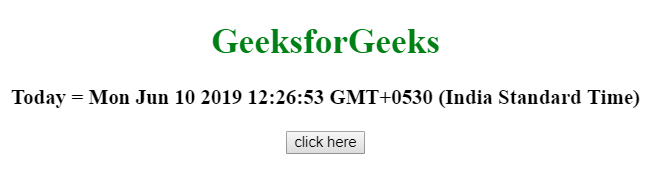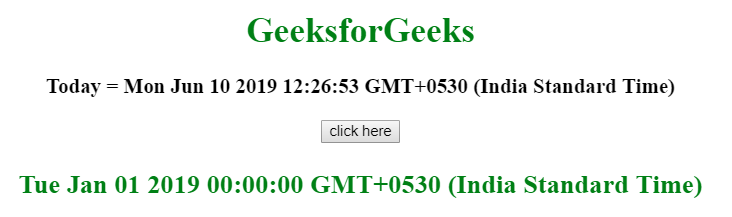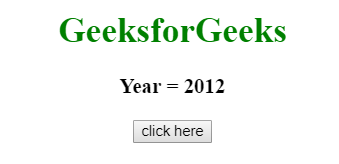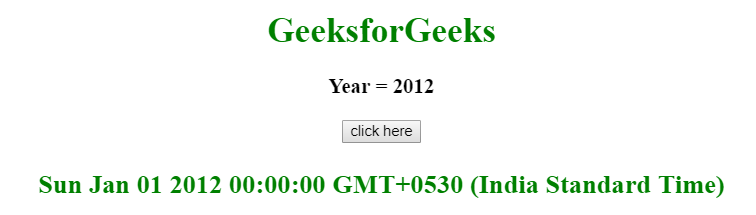# How to get the first day of the year in JavaScript ?

Given a date/year and the task is to get the first day of the year using JavaScript.

Approach 1:

• Use getFullYear() Method to get the year from given date.
• Use the new Date() function to create the new date object using year, month and day.

Example: This example uses the getFullYear() method to get the full year of the current day and then get the first day of that year.

 `  ` `<``html``>  ` `    ``<``head``>  ` `        ``<``title``>  ` `            ``How to get the first day of ` `            ``the year in JavaScript ? ` `        `` ` `    ``  ` `     `  `    ``<``body` `style` `= ``"text-align:center;"``>  ` `         `  `        ``<``h1` `style` `= ``"color:green;"` `>  ` `            ``GeeksForGeeks  ` `        `` ` `         `  `        ``<``p` `id` `= ``"GFG_UP"` `style` `= ` `            ``"font-size: 19px; font-weight: bold;"``> ` `        `` ` `         `  `        ``<``button` `onClick` `= ``"GFG_Fun()"``> ` `            ``click here ` `        `` ` `         `  `        ``<``p` `id` `= ``"GFG_DOWN"` `style` `= ` `            ``"color: green; font-size: 24px; font-weight: bold;"``> ` `        `` ` `         `  `        ``<``script``> ` `            ``var up = document.getElementById('GFG_UP'); ` `            ``var down = document.getElementById('GFG_DOWN'); ` `             `  `            ``var date = new Date(); ` `     `  `            ``up.innerHTML = "Today = "+ date; ` `             `  `            ``function GFG_Fun() { ` `                 `  `                ``// Use Date(year, month, day) function ` `                ``down.innerHTML = new Date(date.getFullYear(), 0, 1); ` `            ``} ` `        ``  ` `    ``  ` `                     `

Output:

• Before clicking on the button:• After clicking on the button:Approach 2:

• Initialize the year to the variable (year = 2012).
• Use new Date() function to create the new date object using year, month and day.

Example: This example uses the year 2012 and then get the first day of that year.

 `  ` `<``html``>  ` `    ``<``head``>  ` `        ``<``title``>  ` `            ``How to get the first day of ` `            ``the year in JavaScript ? ` `        `` ` `    ``  ` `     `  `    ``<``body` `style` `= ``"text-align:center;"``>  ` `         `  `        ``<``h1` `style` `= ``"color:green;"` `>  ` `            ``GeeksForGeeks  ` `        `` ` `         `  `        ``<``p` `id` `= ``"GFG_UP"` `style` `= ` `            ``"font-size: 19px; font-weight: bold;"``> ` `        `` ` `         `  `        ``<``button` `onClick` `= ``"GFG_Fun()"``> ` `            ``click here ` `        `` ` `         `  `        ``<``p` `id` `= ``"GFG_DOWN"` `style` `= ` `            ``"color: green; font-size: 24px; font-weight: bold;"``> ` `        `` ` `         `  `        ``<``script``> ` `            ``var up = document.getElementById('GFG_UP'); ` `            ``var down = document.getElementById('GFG_DOWN'); ` `             `  `            ``// Declare year and initialize it ` `            ``var year = 2012; ` `             `  `            ``up.innerHTML = "Today's year = "+ year; ` `             `  `            ``function GFG_Fun() { ` `                 `  `                ``// Use Date(year, month, day) function ` `                ``// to get the first day of year ` `                ``down.innerHTML = new Date(year, 0, 1); ` `            ``} ` `        ``  ` `    ``  ` `                     `

Output:

• Before clicking on the button:• After clicking on the button:My Personal Notes arrow_drop_upCheck out this Author's contributed articles.

If you like GeeksforGeeks and would like to contribute, you can also write an article using contribute.geeksforgeeks.org or mail your article to contribute@geeksforgeeks.org. See your article appearing on the GeeksforGeeks main page and help other Geeks.

Please Improve this article if you find anything incorrect by clicking on the "Improve Article" button below.